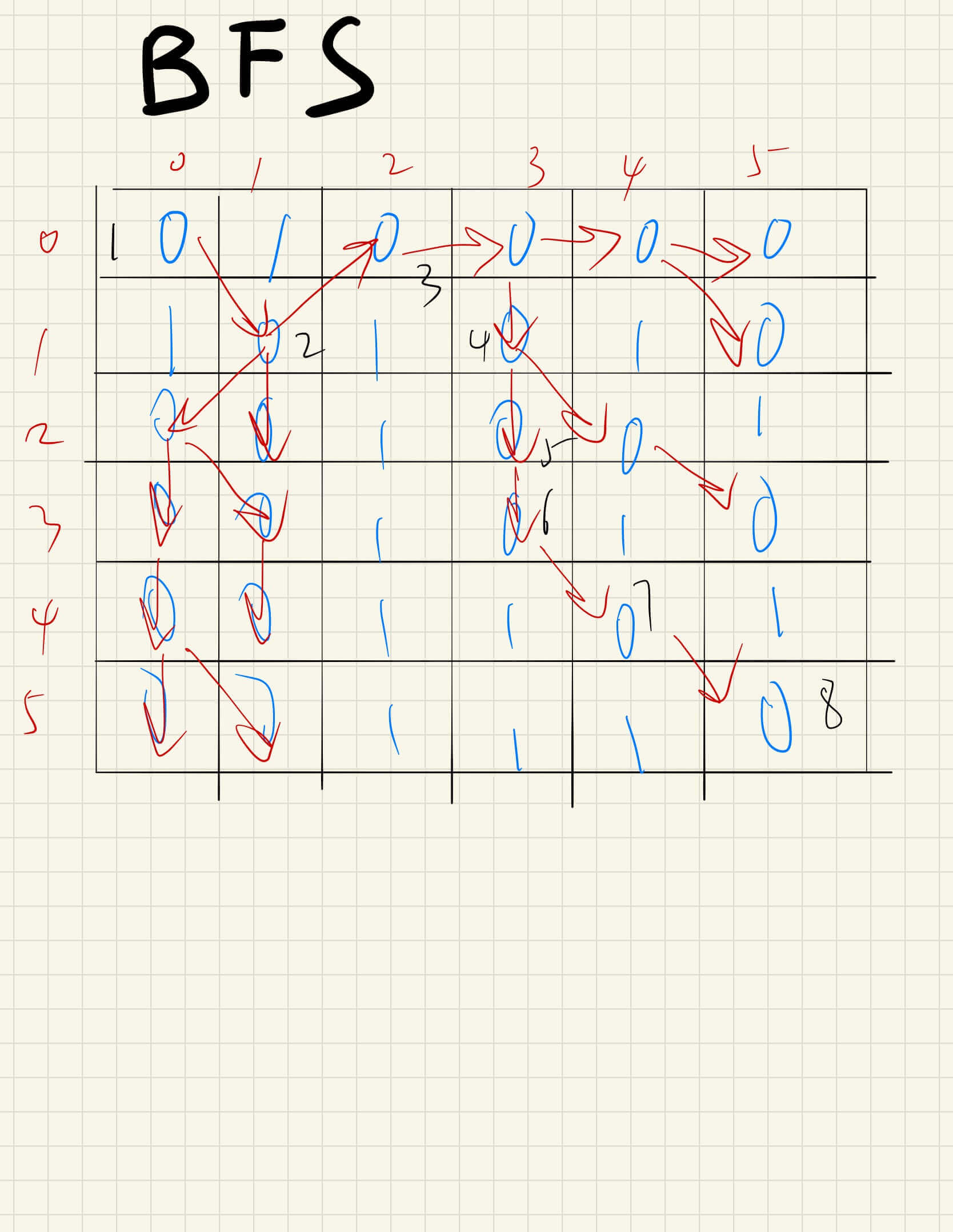ARTS 第十二周
Toc
ARTS Week-12## Algorithm

1091. 二进制矩阵中的最短路径

在一个 N × N 的方形网格中，每个单元格有两种状态：空（0）或者阻塞（1）。

C_1 位于 (0, 0)（即，值为 grid）
C_k 位于 (N-1, N-1)（即，值为 grid[N-1][N-1]）

1 <= grid.length == grid.length <= 100
grid[i][j] 为 0 或 1from collections import deque
class Solution:
def shortestPathBinaryMatrix(self, grid: List[List[int]]) -> int:
n = len(grid)

if grid == 1 or grid[-1][-1] == 1:
return -1

queue = deque([((0,0), 1)])
visited = {(0, 0)}

drs = [-1, 0, 1]
dcs = [-1, 0, 1]

while queue:
cur, step = queue.popleft()
r0, c0 = cur

if r0 == n - 1 and c0 == n - 1:
return step

for dr in drs:
for dc in dcs:
r, c = r0 + dr, c0 + dc

if 0 <= r < n and 0 <= c < n and grid[r][c] == 0 and (r, c) not in visited:
queue.append(((r, c), step + 1))

return -1


## Review

《97 Things Every Programmer Should Know》- Choose Your Tools with Care

## Tip

jupyter lab - jupyter notebook 的升级版，兼容 notebook。

• 可视化文件树
• 支持 ipython console
• 支持 terminal## Share

《迁移到云原生应用架构》中文版

Pivotal 是云原生应用的提出者，并推出了 Pivotal Cloud Foundry 云原生应用平台和 Spring 开源 Java 开发框架，成为云原生应用架构中先驱者和探路者。